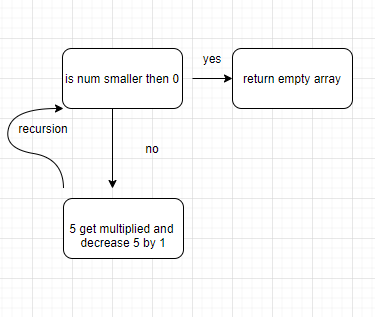# I am stuck with: Factorialize a Number

Tell us what’s happening:So my idea was as can be seen in the diagram above is to substract a number of 5 and multiply it
The only problem I have
is with line 5/6 it takes over which leads to the following error:

SyntaxError: unknown: Identifier ‘countArray’ has already been declared (6:10)
Is there any way I can solve this?

``````
function factorialize(num) {
if (num < 1) {
return [];
} else {
const countArray = factorialize(num - 1);
const countArray = factorialize(num * 1);
countArray.unshift(num);
return countArray;
}

}

factorialize(5);
``````

User Agent is: `Mozilla/5.0 (Windows NT 10.0; Win64; x64) AppleWebKit/537.36 (KHTML, like Gecko) Chrome/86.0.4240.193 Safari/537.36`.

Challenge: Factorialize a Number

Hi @KittyKora. I have no idea why you have chosen that route. Essentially what you want to achieve is something like `n * n - 1 * n - 2 * ... * 1`. Something like:

``````function factorialize(num) {
if (num < 1)  return  1; // base condition
return num * factorialize(num - 1); // recursive condition
}
``````

would suffice.

1 Like

The reason why you are getting an error is because const stands for constant. A constant cannot be reassigned.

2 Likes

Because i find aritimetic’s hard to deal with
when it’s someting simple like
const countArray = factorialize(num - 1);
which only does 3 things i can manage
but things like
return num * factorialize(num - 1); // recursive condition
which does a couple of things is hard
and it’s get confusing